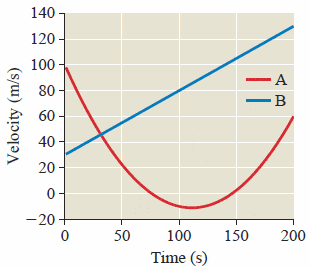# [Motion Problem] When is displacement equal?

The figure below shows a velocity versus time plot for two objects; they are at the same point at
t = 0.## Homework Statement

Do the objects ever have the same displacement? If so, when does that happen?

## Homework Equations

Δd = ((velocityi+ velocityf) / 2) Δt
acceleration = change in velocity / change in time
Δd(A) = 100Δt + 0.5 (accel. Δt^2)
Δd(B) = 30Δt + 0.5 (accel. Δt^2)

## The Attempt at a Solution

Looking at the graph, I can tell that line:B has a constantly changing velocity and therefore a constant acceleration. Line:A is different, it resembles a quadratic and displays the characteristic of a non-constant (time dependent acceleration). I know that the displacement between the two lines will be the same when the sum of initial and final velocity are equal given by my displacement equation.

Could someone please help to guide me towards this solution? It seems incredibly simple but I just can't grasp it. :(

Last edited:

rude man
Homework Helper
Gold Member
You need to use the more fundamental relationship between distance and speed: in this case,
d(t) = ∫v(t)dt. Use that for both curves.

To get a precise numerical answer you have to know what vA(t) is. Looks like a parabola to me, so derive the equation for the A curve. The B curve is obvioulsy a straight line, as you said.

Otherwise you can try to approximate the answer graphically. Not easily, though.

Representing the lines in equations

So I know that the line:B can be graphed with y = mx + b, which I resolved to equal y = 0.5x + 30. For line:A, I am having trouble representing it in an equation form. It appears to be quadratic, therefore it should be represented with y = ax^2 + bx + c. Where c = 100, I equated a to equal 1.6 using the first 50 seconds as a basis. I can't seem to find the solution to the second equation but I can estimate the x-int to be about 75 and 145, with a y-int of 100. What am I doing wrong here?

rude man
Homework Helper
Gold Member
A parabola would be v-v0 = k(t - t0)^2. Try that.
v0 = -10 is pretty obvious but t0 less so. k somewhere around 0.01, t0 around 110 s.